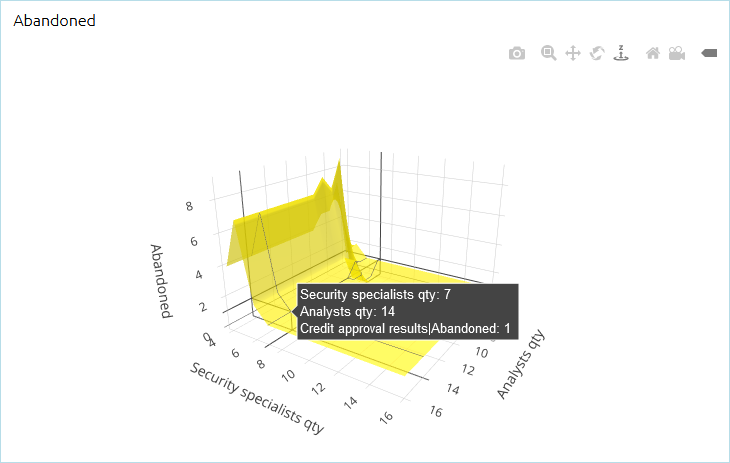AnyLogic

# Output charts for experiments with multiple runs

For experiments that require multiple runs (for example, Monte Carlo, parameter variation, and so on), specific types of output charts are available in AnyLogic Cloud.

## Mean and error plot

Mean and error plot displays the mean value of calculated data and how it varies. A single data point represents the mean value of data and error bars represent the overall data distribution, that is, the margin of error. The size of the error bar represents the confidence level for the calculated mean value: the more lower and upper edges of the error bar deviate from the mean point, the less confident this calculation is.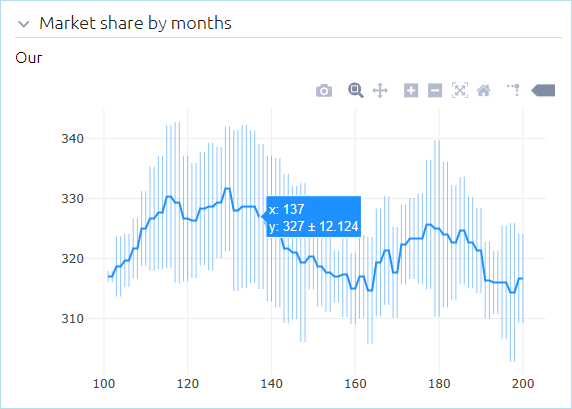## Box plot

Box plot displays probability distribution for sets of data in quartiles:

• the 1st quartile bottom edge (q1) corresponds to the 25th percentile (that is, 25% of the whole values of this data set will fall below this value)
• the 2nd quartile represents the median value
• the 3rd quartile top edge (q3) corresponds to the 75th percentile

Box plot also displays data values that lay outside of the 1st and 3rd quartiles — in the form of vertical segments commonly known as whiskers. The whiskers extend to the maximum and minimum observed values of the data set. Data outliers are plotted as individual points.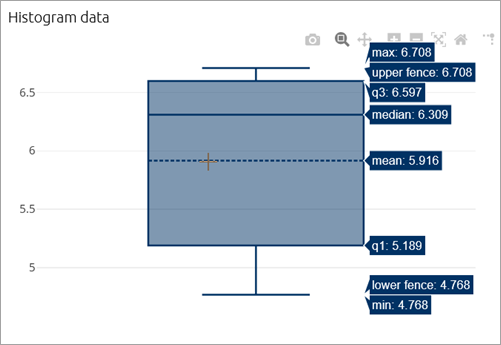## Scatter plot

Scatter diagram represents the dependency between two variables: an independent variable, whose value is changed according to the specified rule and the dependent variable, whose value is calculated according to the changes of independent variable. The values are plotted as a collection of individual points, where the value of independent variable determines the point's position on the X axis, and the value of the dependent variable determines its position on the Y axis.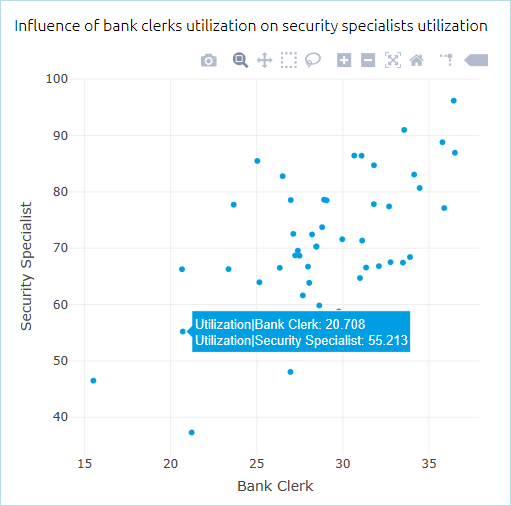## Density plot

Density plot represents the probability distribution of value in a data set. The values of the data set from each run are plotted in a line along the X axis, and after multiple runs the areas of the plot with the highest probability density will overlap. The value along the Y axis represents the probability density of the corresponding value along the X axis.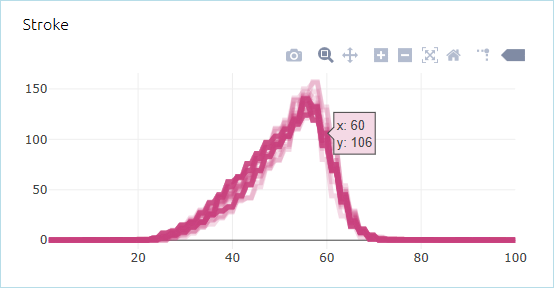## 3D surface graph

3D surface graph is available for the following types of experiments: Variation and Variation with replications.

3D surface graph displays the interaction of two variating parameters and how the values of these parameters (X and Y) affect the output value, shown along the Z axis.